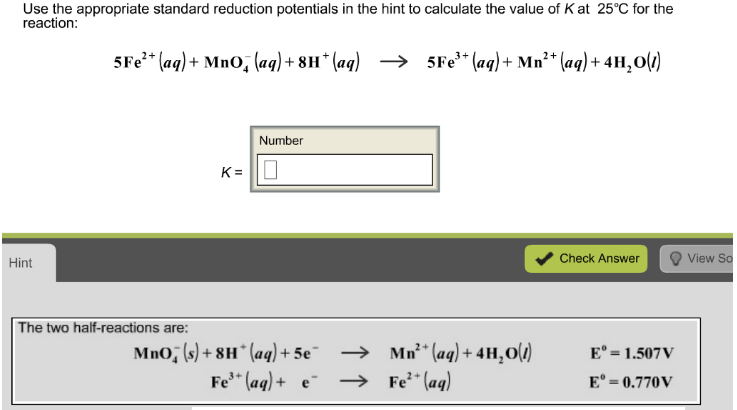# Problem: Use the appropriate standard reduction potentials in the hint to calculate the value of K at 25 °C for the reaction: 5Fe2+(aq) + MnO-4(aq) → 5Fe3+(aq) + Mn2+(aq) + 4H2O(l)

###### FREE Expert Solution
96% (365 ratings)###### Problem Details

Use the appropriate standard reduction potentials in the hint to calculate the value of K at 25 °C for the reaction:

5Fe2+(aq) + MnO-4(aq) → 5Fe3+(aq) + Mn2+(aq) + 4H2O(l)